1800-1023-196

+91-120-4616500

# Methods of Determination of Equivalent Mass

## Methods of Determination of Equivalent Mass

Hydrogen displacement method: The mass of metal which displaces 11200 ml of hydrogen at NTP from an acid, alkali or alcohol is the equivalent mass of the metal.

(a) Equivalent mass of metal

(b) Equivalent mass of metal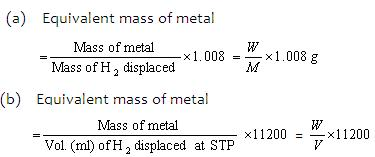This method is useful for metals which can displace hydrogen from acids or can combine with hydrogen (Mg, Zn, Ca, etc.)

(ii) Oxide formation method: The mass of the element which combines with 8 grams of oxygen is the equivalent mass of the element.

(a) Equivalent mass of metal = (Mass of metal/Mass of oxygen)×8

(b) Equivalent mass of metal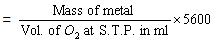(iii) Chloride formation method : The mass of an element which reacts with 35.5 gm. of chlorine is the equivalent mass of that element.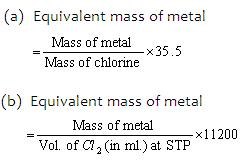(iv) Neutralisation method: (For acids and bases).

Equivalent mass of acid (or base) = W/v×N

Where ,

W = Mass of acid or base in gm.,

V = Vol. of base or acid in litre required for neutralisation

N is Normality of base or acid

(v) Metal displacement method : It is based on the fact that one gm. equivalent of a more electropositive metal displaces one gm equivalent of a less electropositive metal from its salt solution.(vi) Electrolytic method: The quantity of substance that reacts at electrode when 1 faraday of electricity is passed is equal to its gram equivalent mass.

Gram equivalent mass = Electrochemical equivalent 96500

The ratio of masses of two metals deposited by the same quantity of electricity will be in the ratio of their equivalent masses.

W1/W2 = E1/E2

(vii) Double decomposition method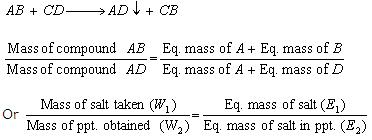(viii) Conversion method: When one compound of a metal is converted to another compound of the same metal, then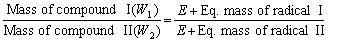(E = Eq. mass of the metal)

(ix) Volatile chloride method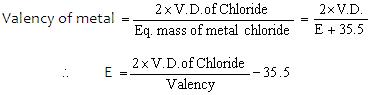## NEET & AIIMS Exam Sample Papers

 AIIMS SAMPLE PAPERS View More NEET SAMPLE PAPERS View More